Mathematical and Physical Journal
for High Schools
Issued by the MATFUND Foundation
 Already signed up? New to KöMaL?

# KöMaL Problems in Physics, April 2014

Show/hide problems of signs:## Problems with sign 'M'

Deadline expired on May 12, 2014.

M. 341. Beat several egg whites until stiff. Measure by what factor did the volume of the egg white increase. What is the average volume of the bubbles? Estimate the number of bubbles.

(6 pont)

statistics## Problems with sign 'P'

Deadline expired on May 12, 2014.

P. 4627. Holding a blown-up balloon in our hands, after some time we feel that the balloon heats our hand. How can it be?

(3 pont)

solution (in Hungarian), statistics

P. 4628. A car travelling on road 4 had an average speed of 75 km/h between the cities Szolnok and Kisújszállás, which are at a distance of 50 km, and between Kisújszállás and Karcag, which are at a distance of 10 km, its average speed was 80 km/h. Can this car cover the total distance from Szolnok to Debrecen in 1.5 hours if in the remaining 64 km between Karcag and Debrecen it is not allowed to exceed the speed of 90 km/h?

(3 pont)

solution (in Hungarian), statistics

P. 4629. An object is left to move along a horizontal surface, on which friction is not negligible. At one moment its kinetic energy is 900 J, and 2 s later it is only 400 J, while the object covers a distance of $\displaystyle \ell$.

$\displaystyle a)$ By what factor will the further distance until the object stops be greater than the distance $\displaystyle \ell$?

$\displaystyle b)$ How long does it take to cover this distance?

(4 pont)

solution (in Hungarian), statistics

P. 4630. A sack of sand is lying on a light carpet on a horizontal surface. With the help of the carpet the sack of mass $\displaystyle M$ is to be pulled from a smooth surface to a rough one. How much work is done if the coefficients of kinetic friction between the surfaces and the carpet are: $\displaystyle \mu_1$, and $\displaystyle \mu_2$? The weight of the sack is not uniform, but we know that the centre of mass of the sack is at a distance of $\displaystyle s_1$, and $\displaystyle s_2$ from the ends of the bag.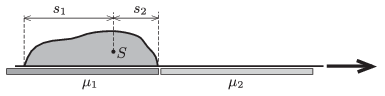(5 pont)

solution (in Hungarian), statistics

P. 4631. A step ladder is placed to the smooth horizontal surface of ice. The cross section of the ladder is the same everywhere and the angle between its legs is $\displaystyle \alpha=60^\circ$. The hinge which connects the two legs of mass 5 kg and of length of 2 m is frictionless. The thin, initially tight safety rope breaks.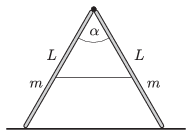$\displaystyle a)$ What is the angle between the legs of the ladder, when the hinge does not exert any force?

$\displaystyle b)$ What is the force exerted on the ice by the ladder at this moment?

$\displaystyle c)$ At what speed does the hinge hit the ice?

(5 pont)

solution (in Hungarian), statistics

P. 4632. A sample of low pressure gas of given mass, is taken from state $\displaystyle A$ to state $\displaystyle B$, along the process $\displaystyle AB$ as shown in the figure. How much work is done?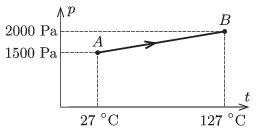(4 pont)

solution (in Hungarian), statistics

P. 4633. The working substance of a heat engine is a sample of monatomic ideal gas of specific mass. The gas may be taken through the cyclic process $\displaystyle ABCA$ or $\displaystyle ADEA$ as shown in the figure. What is the ratio of the two efficiencies?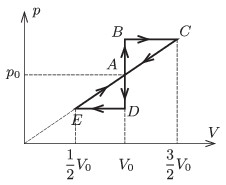(5 pont)

solution (in Hungarian), statistics

P. 4634. At two vertices of a regular hexagon of side $\displaystyle a=12$ cm, there are two charges of charge $\displaystyle Q=6.4\cdot10^{-8}$ C, and at a third vertex there is a charge of $\displaystyle -Q$, as shown in the figure. What is the direction and the magnitude of the electric field strength at the centre of the hexagon and at those vertices at which there is no charge?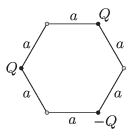(4 pont)

solution (in Hungarian), statistics

P. 4635. What is the resistance of the resistor R connected into the circuit shown in the figure, if the voltage across the condenser is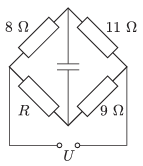$\displaystyle a)$ $\displaystyle U_{C}=0$;

$\displaystyle b)$ $\displaystyle U_{C}=\frac{U}{2}$?

(4 pont)

solution (in Hungarian), statistics

P. 4636. The width of the slits in a diffraction grating is one-$\displaystyle n$-th of the grating constant. The diffraction grating of grating constant $\displaystyle d$ is illuminated perpendicularly by monochromatic laser light of wavelength $\displaystyle \lambda$, and the diffraction pattern is examined on a screen at a distance of $\displaystyle L\gg d$. Give the position of the different order diffraction lines on the screen.

(5 pont)

solution (in Hungarian), statistics

P. 4637. A chocolate bunny wrapped into aluminium foil is suspended by a piece of insulating chord and is charged. The bunny slowly looses its charge due to the conducting ability of air. During the discharging process, what is the magnetic field around the bunny like? (It can be assumed that the conducting ability of air is the same everywhere.)(6 pont)

solution (in Hungarian), statistics